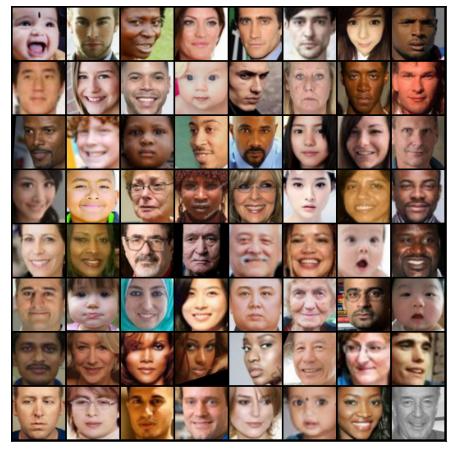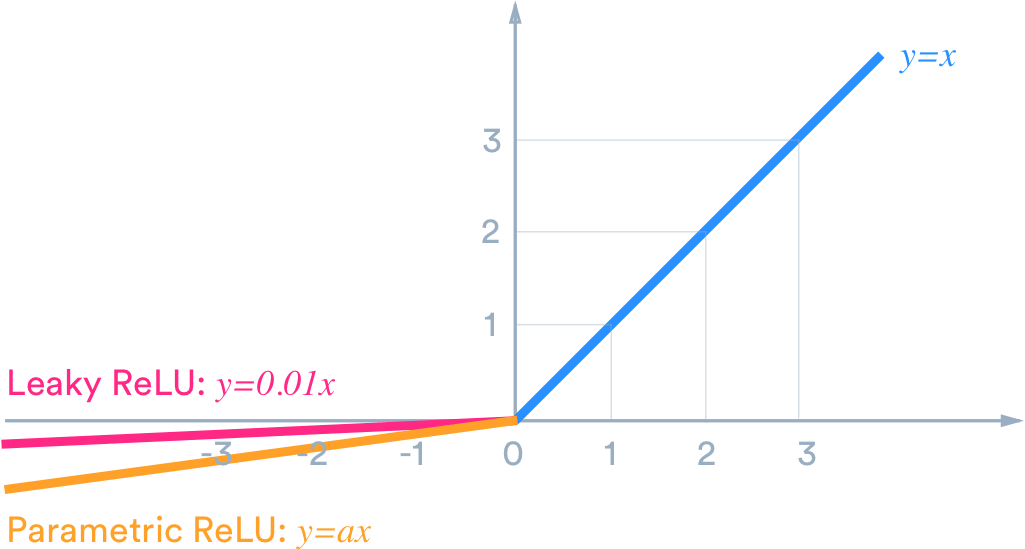Learn data science and machine learning by building real-world projects on Jovian
In [ ]:
``!pip install jovian --upgrade --quiet``

## FakeFaceGAN

In this project, I am trying to Generate fake human faces from original human faces using General Adversial Networks (GANs) in Pytorch. Which has been taught us by our instructor of this course Aakash N S . Who made this course very easy to understand by explaining each and every line of codes and concepts in both languages Hindi and English. he created interest in me towards artficial intlelligence and machine learning. Looking farword to learn more about AI and ML by his upcoming courses.

Generative modeling is an unsupervised learning task in machine learning that involves automatically discovering and learning the regularities or patterns in input data in such a way that the model can be used to generate or output new examples that plausibly could have been drawn from the original dataset. - Source

While there are many approaches used for generative modeling, a Generative Adversarial Network takes the following approach:There are two neural networks: a Generator and a Discriminator. The generator generates a "fake" sample given a random vector/matrix, and the discriminator attempts to detect whether a given sample is "real" (picked from the training data) or "fake" (generated by the generator). Training happens in tandem: we train the discriminator for a few epochs, then train the generator for a few epochs, and repeat. This way both the generator and the discriminator get better at doing their jobs.

GANs however, can be notoriously difficult to train, and are extremely sensitive to hyperparameters, activation functions and regularization. In this project, we'll train a GAN to generate fake human faces from original human faces.

In [ ]:
``project_name = 'GANS-project'``

## Dataset

I am using the utk-face-cropped dataset from kaggle, which consists of over 24,000 human faces. Note that generative modeling is an unsupervised learning task, so the images do not have any labels.

Using the `opendatasets` library to download the dataset from Kaggle. `opendatasets` uses the Kaggle Official API for downloading datasets from Kaggle.

In [ ]:
``!pip install opendatasets --upgrade --quiet``
In [ ]:
``````import opendatasets as od

dataset_url = 'https://www.kaggle.com/abhikjha/utk-face-cropped'
``````
` 0%| | 0.00/232M [00:00<?, ?B/s]`
```Downloading utk-face-cropped.zip to ./utk-face-cropped ```
```100%|██████████| 232M/232M [00:02<00:00, 107MB/s] ```
``` ```
In [ ]:
``````import os

DATA_DIR = './utk-face-cropped'
print(os.listdir(DATA_DIR))
``````
```['utkcropped'] ```

The dataset has a single folder called 'utkcropped' which contains 24,000 images in jpg format.which are well cropped and aligned.

In [ ]:
``print(os.listdir(DATA_DIR+'/utkcropped')[:10])``
```['26_1_4_20170117154131789.jpg.chip.jpg', '28_0_1_20170113155330532.jpg.chip.jpg', '4_1_0_20170104005821656.jpg.chip.jpg', '26_0_1_20170113151702303.jpg.chip.jpg', '55_1_3_20170109142107754.jpg.chip.jpg', '35_0_0_20170117175758802.jpg.chip.jpg', '51_0_0_20170117190825002.jpg.chip.jpg', '33_1_1_20170113005238142.jpg.chip.jpg', '1_1_0_20161219161636014.jpg.chip.jpg', '31_0_1_20170116192548730.jpg.chip.jpg'] ```

Loading this dataset using the `ImageFolder` class from `torchvision`. We will also resize and crop the images to 64x64 px, and normalize the pixel values with a mean & standard deviation of 0.5 for each channel. This will ensure that pixel values are in the range `(-1, 1)`, which is more convenient for training the discriminator. We will also create a data loader to load the data in batches.

In [ ]:
``````from torch.utils.data import DataLoader
from torchvision.datasets import ImageFolder
import torchvision.transforms as T``````
In [ ]:
``````image_size = 64
batch_size = 128
stats = (0.5, 0.5, 0.5), (0.5, 0.5, 0.5)``````
In [ ]:
``````train_ds = ImageFolder(DATA_DIR, transform=T.Compose([
T.Resize(image_size),
T.CenterCrop(image_size),
T.ToTensor(),
T.Normalize(*stats)]))

train_dl = DataLoader(train_ds, batch_size, shuffle=True, num_workers=3, pin_memory=True)``````

Creating helper functions to denormalize the image tensors and display some sample images in grid from a training batch.

In [ ]:
``````import torch
from torchvision.utils import make_grid
import matplotlib.pyplot as plt
%matplotlib inline
``````
In [ ]:
``````def denorm(img_tensors):
return img_tensors * stats + stats``````
In [ ]:
``````def show_images(utkcropped, nmax=64):
fig, ax = plt.subplots(figsize=(8, 8))
ax.set_xticks([]); ax.set_yticks([])
ax.imshow(make_grid(denorm(utkcropped.detach()[:nmax]), nrow=8).permute(1, 2, 0))

def show_batch(dl, nmax=64):
for utkcropped, _ in dl:
show_images(utkcropped, nmax)
break``````
In [ ]:
``show_batch(train_dl)``In [ ]:
``!pip install jovian --upgrade --quiet``
In [ ]:
``jovian.commit(project=project_name, environment=None)``
```[jovian] Detected Colab notebook... [jovian] Please enter your API key ( from https://jovian.ai/ ): API KEY: ·········· [jovian] Uploading colab notebook to Jovian... [jovian] Committed successfully! https://jovian.ai/mishalkumar/gans-project ```
Out[]:
``'https://jovian.ai/mishalkumar/gans-project'``

### Using a GPU

To seamlessly use a GPU, if one is available, we define a couple of helper functions (`get_default_device` & `to_device`) and a helper class `DeviceDataLoader` to move our model & data to the GPU, if one is available.

In [ ]:
``````def get_default_device():
"""Pick GPU if available, else CPU"""
if torch.cuda.is_available():
else:

def to_device(data, device):
"""Move tensor(s) to chosen device"""
if isinstance(data, (list,tuple)):
return [to_device(x, device) for x in data]
return data.to(device, non_blocking=True)

"""Wrap a dataloader to move data to a device"""
def __init__(self, dl, device):
self.dl = dl
self.device = device

def __iter__(self):
"""Yield a batch of data after moving it to device"""
for b in self.dl:
yield to_device(b, self.device)

def __len__(self):
"""Number of batches"""
return len(self.dl)``````
In [ ]:
``````device = get_default_device()
device
``````
Out[]:
``device(type='cuda')``

We can now move our training data loader using `DeviceDataLoader` for automatically transferring batches of data to the GPU (if available).

In [ ]:
``train_dl = DeviceDataLoader(train_dl, device)``

### Discriminator Network

The discriminator takes an image as input, and tries to classify it as "real" or "generated". In this sense, it's like any other neural network. We'll use a convolutional neural networks (CNN) which outputs a single number output for every image. We'll use stride of 2 to progressively reduce the size of the output feature map.In [ ]:
``import torch.nn as nn``
In [ ]:
``````discriminator = nn.Sequential(
# in: 3 x 64 x 64

nn.Conv2d(3, 64, kernel_size=4, stride=2, padding=1, bias=False),
nn.BatchNorm2d(64),
nn.LeakyReLU(0.2, inplace=True),
# out: 64 x 32 x 32

nn.Conv2d(64, 128, kernel_size=4, stride=2, padding=1, bias=False),
nn.BatchNorm2d(128),
nn.LeakyReLU(0.2, inplace=True),
# out: 128 x 16 x 16

nn.Conv2d(128, 256, kernel_size=4, stride=2, padding=1, bias=False),
nn.BatchNorm2d(256),
nn.LeakyReLU(0.2, inplace=True),
# out: 256 x 8 x 8

nn.Conv2d(256, 512, kernel_size=4, stride=2, padding=1, bias=False),
nn.BatchNorm2d(512),
nn.LeakyReLU(0.2, inplace=True),
# out: 512 x 4 x 4

nn.Conv2d(512, 1, kernel_size=4, stride=1, padding=0, bias=False),
# out: 1 x 1 x 1

nn.Flatten(),
nn.Sigmoid())
``````

Note that we're using the Leaky ReLU activation for the discriminator.Different from the regular ReLU function, Leaky ReLU allows the pass of a small gradient signal for negative values. As a result, it makes the gradients from the discriminator flows stronger into the generator. Instead of passing a gradient (slope) of 0 in the back-prop pass, it passes a small negative gradient. - Source

Just like any other binary classification model, the output of the discriminator is a single number between 0 and 1, which can be interpreted as the probability of the input image being real i.e. picked from the original dataset.

Let's move the discriminator model to the chosen device.

In [ ]:
``discriminator = to_device(discriminator, device)``

### Generator Network

The input to the generator is typically a vector or a matrix of random numbers (referred to as a latent tensor) which is used as a seed for generating an image. The generator will convert a latent tensor of shape `(128, 1, 1)` into an image tensor of shape `3 x 28 x 28`. To achive this, we'll use the `ConvTranspose2d` layer from PyTorch, which is performs to as a transposed convolution (also referred to as a deconvolution). Learn moreIn [ ]:
``latent_size = 128``
In [ ]:
``````generator = nn.Sequential(
# in: latent_size x 1 x 1

nn.ConvTranspose2d(latent_size, 512, kernel_size=4, stride=1, padding=0, bias=False),
nn.BatchNorm2d(512),
nn.ReLU(True),
# out: 512 x 4 x 4

nn.ConvTranspose2d(512, 256, kernel_size=4, stride=2, padding=1, bias=False),
nn.BatchNorm2d(256),
nn.ReLU(True),
# out: 256 x 8 x 8

nn.ConvTranspose2d(256, 128, kernel_size=4, stride=2, padding=1, bias=False),
nn.BatchNorm2d(128),
nn.ReLU(True),
# out: 128 x 16 x 16

nn.ConvTranspose2d(128, 64, kernel_size=4, stride=2, padding=1, bias=False),
nn.BatchNorm2d(64),
nn.ReLU(True),
# out: 64 x 32 x 32

nn.ConvTranspose2d(64, 3, kernel_size=4, stride=2, padding=1, bias=False),
nn.Tanh()
# out: 3 x 64 x 64
)
``````

We use the TanH activation function for the output layer of the generator.Note that since the outputs of the TanH activation lie in the range `[-1,1]`, we have applied the similar transformation to the images in the training dataset. Let's generate some outputs using the generator and view them as images by transforming and denormalizing the output.

In [ ]:
``````xb = torch.randn(batch_size, latent_size, 1, 1) # random latent tensors
fake_images = generator(xb)
print(fake_images.shape)
show_images(fake_images)
``````
```torch.Size([128, 3, 64, 64]) ```